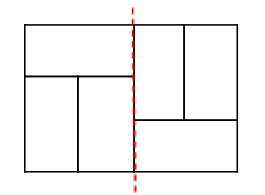#### Question: [Problem E]

Followed by n-1 lines, each contains two integers u and v (1≤ u, v ≤ n), which means it takes one hour to go from level u to level v.

it means that we can't move from v to u!

it should've been "from level u to level v or vice versa".
fixed in the problem statement.

#### Question: [General]

Extension?

The time has been extended for 15 minutes to compensate the long judge queue and problem E ambiguity.

#### Question: [Problem E]

Another test case?

input:

1
7
1 2
2 3
3 4
4 5
5 6
6 7

output:
11

hint: the shortcut should connect to 5. d(1, 4) becomes 2.
adding a shortcut changes the gameplay.

#### Question: [Problem E]

Clarification

in Problem E, you have to add exactly one edge from node 1 to another node in the tree, and print the total minimized distance from the root to all the nodes in the graph, not just to the leaves of the graph.

```1 -- 2 -- 3 -- 4
|
+ -- 5
|
+ -- 6
```

```+---------+
|         |
1 -- 2 -- 3 -- 4
|
+ -- 5
|
+ -- 6
```

then, SUM distance(1, v) for all v in the graph becomes minimized:
d(1, 1)+d(1, 2)+d(1, 3)+d(1, 4)+d(1, 5)+d(1, 6)
0+1+1+2+2+2 = 8

#### Question: [General]

Judge Queue

Please be patient while the problem C gets rejudged.
The queue will be empty soon!

#### Question: [Problem C]

Time Limit

The time limit for problem C has been adjusted. All the TLE submissions are getting rejudged.
We recommend use fast IO functions to read the input.

#### Question: [Problem E]

can you give a simple explanation for problem E sample input ?
it doesnt make sense !

For the first test, the short cut should be to the 3rd level.
So, we would have:
1->2 = 1
1->3 = 1
1->3->4 = 2
1->3->5 = 2
1->3->6 = 2